CONIC SECTIONS - THE ELLIPSE

Example 1:

Find the coordinates of the center, the vertices, and foci of the ellipse given by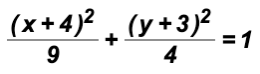.

This equation is almost in standard form. All we have to do is the following:where h =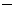4, k =3, a = 3 (from larger denominator), and b = 2

Now, we can find the requested information as follows:

Coordinates of the Center (h, k)

(4,3)

Coordinates of the Vertices (h + a, k) and (ha, k)

(4 + 3,3) = (1,3)

and (43,3) = (7,3)

Coordinates of the Foci (h + c, k) and (hc, k), where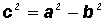Since a = 3 and b = 2 , then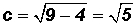.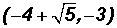andExample 2:

Find the coordinates of the center, vertices, and foci of the ellipse given by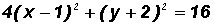.

First, we will convert the equation of the ellipse to standard form.

It is eitheror, where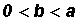First, we will divide both sides of the equation by 16 to get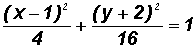We can now write the standard form of the given elliptic equation as follows: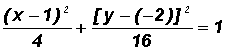where h = 1, k =2, a = 4 (from larger denominator), and b = 2

Now, we can find the requested information as follows:

Coordinates of the Center (h, k)

(1,2)

Coordinates of the Vertices (h, k + a) and (h, ka)

(1,2 + 4) and (1,24)

(1, 2) and (1,6)

Coordinates of the Foci (h, k + c) and (h, kc), whereSince a = 4 and b = 2, then.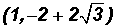and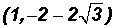Example 3:

Find the coordinates of the center, vertices, and foci of the ellipse given by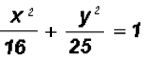.

First, we will convert the equation of the ellipse to standard form, where.

NOTE: The center (h, k) of the given ellipse is at (0, 0) because there is only an x2-term and a y2-term. Therefore, we can writewhere h = 0, k = 0, a = 5 (from larger denominator), and b = 4

Now, we can find the requested information as follows:

Coordinates of the Center (h, k)

(0, 0)

Coordinates of the Vertices (h, k + a) and (h, ka)

(0, 0 + 5)and (0, 05)

(0, 5) and (0,5)

Coordinates of the Foci (h, k + c) and (h, kc), whereSince a = 5 and b = 4, then.

(0, 0 + 3)and (0, 03)

(0, 3) and (0,3)

Example 4:

Find the coordinates of the center, vertices, and foci of the ellipse given by.

First, we will convert the equation of the ellipse to standard form.

It is eitheror, whereFirst, we will divide both sides of the equation by 36 to get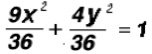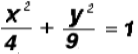We can now write the standard form of the given elliptic equation as follows:where h = 0, k = 0, a = 3 (from larger denominator), and b = 2

Now, we can find the requested information as follows:

Coordinates of the Center (h, k)

(0, 0)

Coordinates of the Vertices (h, k + a) and (h, ka)

(0, 0 + 3) and (0, 03)

(0, 3) and (0,3)

Coordinates of the Foci (h, k + c) and (h, kc), whereSince a = 3 and b = 2, then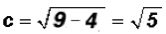.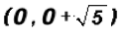and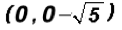and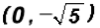Example 5:

Match the names hyperbola, ellipse, parabola, and circle to the following conic equations.

a.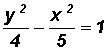We must recognize this as a hyperbola because of its general characteristics. There is a squared x and y-term and one of them is negative. It is actually a hyperbola with center at the origin.

b.We must recognize this as an ellipse because of its general characteristics. There is a squared x and y-term and both are positive. Also, the squared terms have different denominators.

c.We must recognize this as an ellipse because of its general characteristics. There is a squared x and y-term and both are positive. Also, the squared terms have different coefficients (or denominators).

e.We must recognize this as a circle because of its general characteristics. There is a squared x and y-term and both are positive. Also, the squared terms have the same denominators.

f.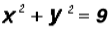We must recognize this as a circle because of its general characteristics. There is a squared x and y-term and both are positive. Also, the squared terms have the same coefficients (or denominators), namely 1.

d.We must recognize this as the standard form of a parabola because of its general characteristics. There is only one squared term, namely y2.